## Tamilnadu Samacheer Kalvi 11th Maths Solutions Chapter 10 Differentiability and Methods of Differentiation Ex 10.1

Question 1.
Find the derivatives of the following functions using first principle.
(i) f(x) = 6
Solution:
Given f(x) = 6
f(x + h) = 6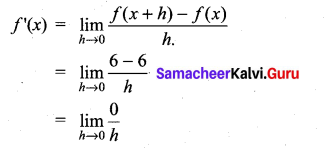[h → 0 means h is very nears to zero from left to right but not zero]

(ii) f(x) = -4x + 7
Solution:
Given f(x) = -4x + 7
f(x + h) = -4(x + h) + 7
= -4x – 4h + 7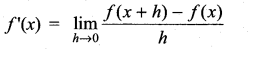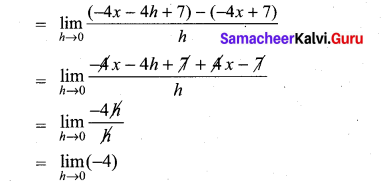(iii) f(x) = -x2 + 2
Given f(x) = -x2 + 2
f(x + h) = -(x + h)2 + 2
= -x2 – h2 – 2xh + 2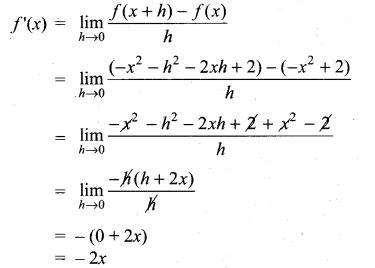Question 2.
Find the derivatives from the left and from the right at x = 1 (if they exist) of the following functions. Are the functions differentiable at x = 1?
(i) f(x) = |x – 1|
Solution: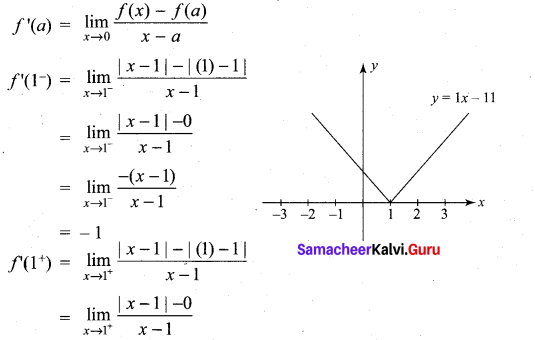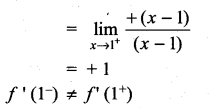f'(1) does not exist
∴ ‘f’ is not differentiable at x = 1.

(ii) f(x) = $$\sqrt{1-x^{2}}$$
Solution: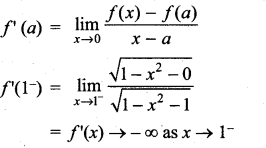∴ ‘f’ is not differentiable at x = 1.

(iii)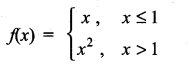Solution: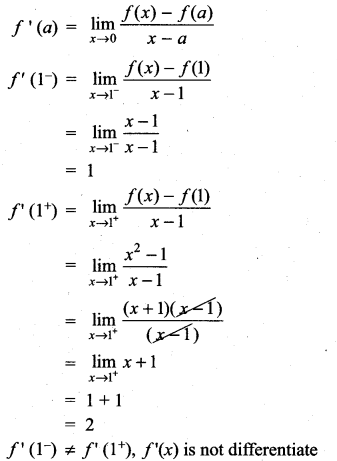‘f’ is not differentiable at x = 1Question 3.
Determine whether the following functions is differentiable at the indicated values.
(i) f(x) = x |x| at x = 0
Solution: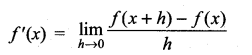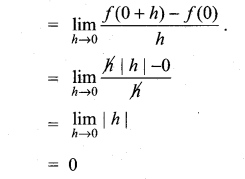Limits exists
Hence ‘f’ is differentiable at x = 0.

(ii) f(x) = |x2 – 1| at x = 1
Solution: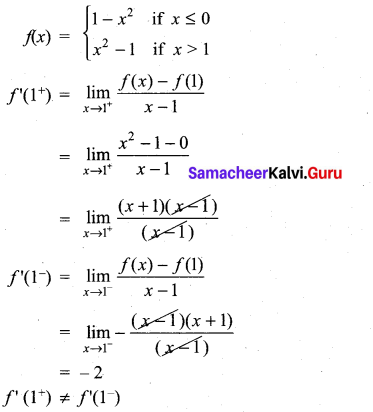f(x) is not differentiable at x = 1.

(iii) f(x) = |x| + |x – 1| at x = 0, 1
Solution: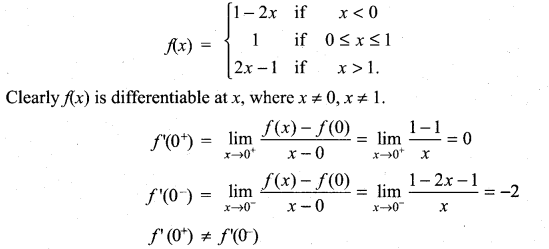∴ f(x) is not differentiable at x = 0.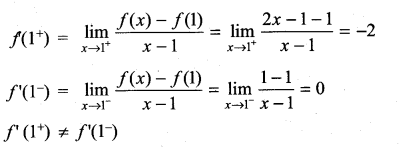∴ f(x) is not differentiable at x = 1.

(iv) f(x) = sin |x| at x = 0
Solution: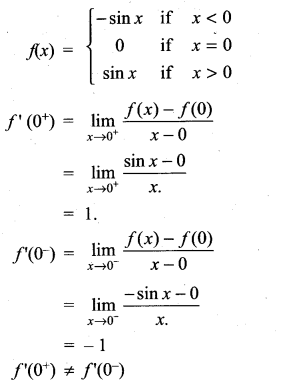∴ f(x) is not differentiable at x = 0.

Question 4.
Show that the following functions are not differentiable at the indicated value of x.
(i)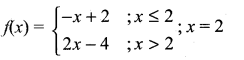Solution: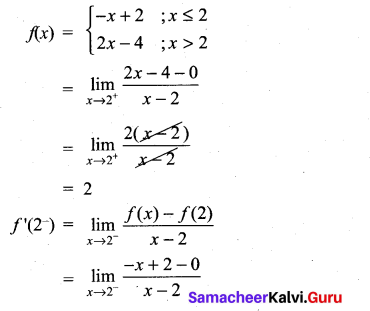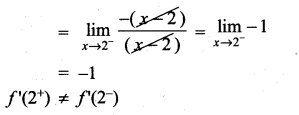f(x) is not differentiable at x = 2.

(ii)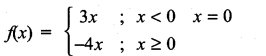Solution: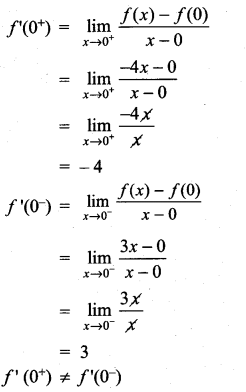f(x) is not differentiable at x = 0.Question 5.
The graph off is shown below. State with reasons that x values (the numbers), at which f is not differentiable.
Solution: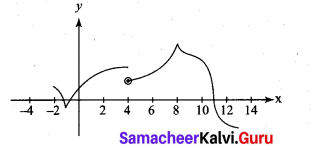(i) at x = – 1 and x = 8. The graph ‘ is not differentiable since ‘ has vertical tangent at x = -1 and x = 8(also At x = -1. The graph has shape edge v] and at x = 8;The graph has shape peak ^]
(ii) At x = 4: The graph f is not differentiable, since at x =4. The graph f’ is not continuous.
(iii) At x = 11; The graph f’ is not differentiable, since at x = 11. The tangent line of the graph is perpendicular.

Question 6.
If f(x) = |x + 100| + x2, test whether f’ (-100) exists.
Solution:
f(x) = |x + 100| + x2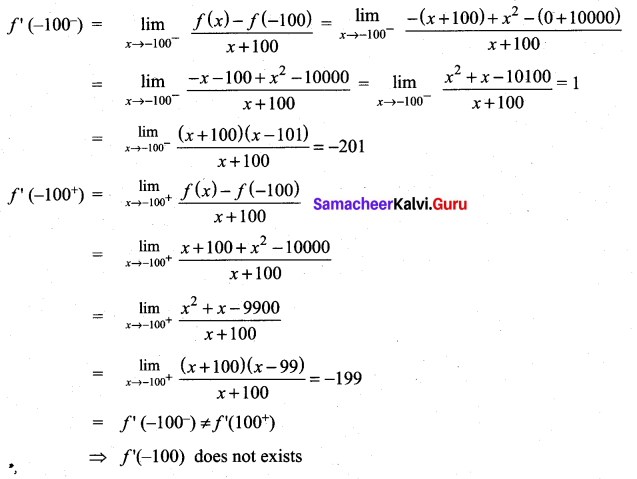Question 7.
Examine the differentiability of functions in R by drawing the diagrams.
(i) |sin x|
Solution: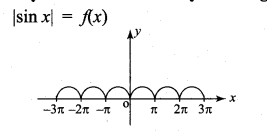Limit exist and continuous for all x ∈ R clearly, differentiable at R — {nπ n ∈ z) Not differentiable at x = nπ , n ∈ z.

(ii) |cos x|
Solution: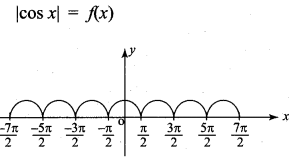Limit exist and continuous for all x ∈ R clearly, differentiable at R {(2n + 1)π/2/n ∈ z} Not differentiable at x = (2n + 1) $$\frac{\pi}{2}$$, n ∈ Z.

### Samacheer Kalvi 11th Maths Solutions Chapter 10 Differentiability and Methods of Differentiation Ex 10.1 Additional Questions

Question 1.
Is the function f(x) = |x| differentiable at the origin. Justify your answer.
Solution: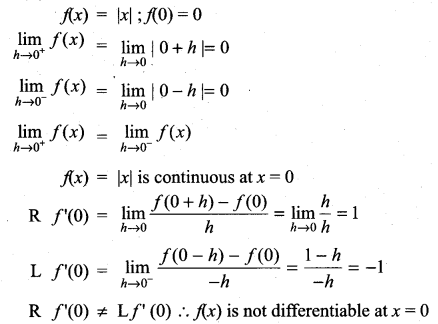Question 2.
Discuss the differentiability of the functions: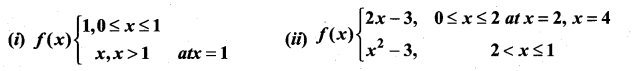Solution: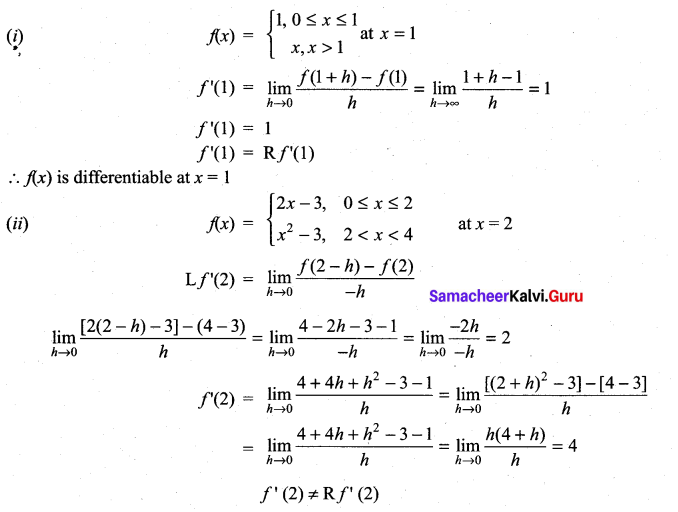∴ f(2) is not differentiable at x = 2. Similarly, it can be proved for x = 4.